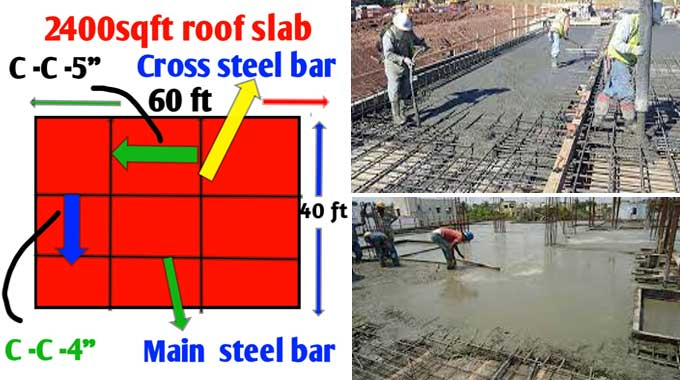# Number of Cement Bags required for a 2400 sq ft RCC Roof SlabCement is one of the most crucial components of concrete; usually, it is mixed in concrete for its adhesive and binding nature consisting of fine and coarse aggregate. A tiny amount of lime is also added to the concrete mixture to escalate the strength and usability of the concrete.

Different grades of concrete, such as M20, M25, M30, are used for casting RCC flat roof slabs. Here, we will use a nominal mix ratio of 1:2:3 (1 pert cement gets mixed up with 2 part sand and 3 part aggregate). The prepared mixture has a compressive strength of 3000 PSI.

For residential buildings, roof slabs are generally kept about 4 inches thick at general standard and 5 inches thick for commercial buildings. This article calculates the cement requirement for RCC roof slabs at 4 inches and 5 inches thick.

The procedure of laying and placing the concrete mix horizontally and structure over brickwork is known as roof slab casting. The main bar and crossbar of the roof slab reinforcement spread over the roof slab's shuttering sections, and then we pour a concrete mix of M20 or M25 grade concrete.

### Cement bags required for 2400 sqft roof slab

Roofing slab casting is the process of laying and placing concrete mix horizontally over brickwork.

The cast of roof slab reinforcement main bar and the crossbar is distributed over shuttering areas of the roof slab, followed by pouring a concrete mix of M20 or M25 grade of concrete. This is called roof slab casting.

Usually, a bag of Portland cement weighed around 50 kg, and its density is 1440 kg/m3.

### Calculating the amount of cement bags required for 2400 sq ft roof slab at 5 inches thick

1. Wet volume of concrete calculated as follows:-1) thickness in feet = 5/ 12 = 0.416 feet, 2) wet volume of concrete = 2400 sq ft × 0.416 feet = 998 cubic feet.

2. Volume of dry concrete = 998 cubic feet × 1.54 = 1537 cubic feet. Thus, a 2400 sq ft RCC roof slab will require 1537 cubic feet of dry concrete.

3. Calculate cement for 2400 square feet of roof slab:

a. Cement quantity in cubic feet =1/6 × dry volume of concrete, 1537 ÷6= 256 cubic feet approximately.

b. Cement aggregate in cubic meters = the volume of concrete in cubic feet ÷ 35.32

c. Quantity of cement in kg = volume of cement in m3 * 1440 kg/m3 = 7.25 × 1440 = 10440 kg approximately.

d. Bags of cement would equal cement quantity in kg ÷ 50 kg = 10440 * 50 = 209 bags approximately.

### Summary

In general, we will need 209 bags of cement (approximately 10,440 kg) to cast a 2400-square-foot roof slab with a 5-inch thickness, which is about 7.25 cubic meters (256 cubic feet) using a 1:3 (cement: sand: aggregate) mix to achieve 3000 psi in 28 days. Therefore, 209 bags of cement are required for a 2400 sq ft RCC roof slab that is 5 inches thick.

### Calculating the amount of cement bags required for 2400 sq ft roof slab at 4 inches thick

1. Wet volume of concrete calculated as follows:-1) thickness in feet = 4/ 12 = 0.33 feet, 2) wet volume of concrete = 2400 sq ft × 0.33 feet = 792 cubic feet.

2. Volume of dry concrete = 792 cubic feet × 1.54 = 1220 cubic feet. Therefore, a 2400 square foot RCC roof slab with a 5-inch thickness will require 1220 cubic feet of dry concrete.

3. Calculate cement for 2400 square feet of roof slab:

To learn more, watch the following video tutorial.

Video Source: Civil Engineers

a. cement quantity in cubic feet =1/6 × dry volume of concrete, 1220÷6= 204 cubic feet approximately.

b. Cement aggregate in cubic meters = the volume of concrete in cubic feet ÷ 35.32, (204 ÷ 35.32 = 5.75 cubic meters approximately).

c. Quantity of cement in kg = volume of cement in m3 * 1440kg/m3 = 5.75 × 1440 = 8280 kg approximately.

d. Bags of cement would equal cement quantity in kg ÷ 50 kg = 8228 ÷ 50 = 166 bags approximately.

### Summary

Generally, to gain 3000 Psi compressive strength of concrete in 28 days using a 1:2:3 (cement: sand: aggregate) mix to achieve a 2490 sq ft RCC roof slab casting at 4 inches thick, we will need 166 bags (around 8280 kg) of cement which is approximately the same as 204 cubic feet or 5.75 cubic meters of adhesive by using 1:2:3 (cement: sand: aggregate) mix.

Therefore, 166 bags of cement are needed for a 2400 sq ft RCC roof slab that is 4 inches thick.